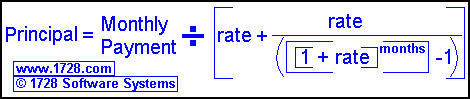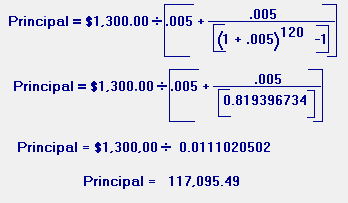Loan Payment Formula - PrincipalWhere rate = Annual Percentage Rate divided by 1200
For example, an 8 % annual rate becomes .0066666666666666...

 Here, the loan formula has been solved for principal. The local bank can arrange for us to have a 10 year loan at 6 per cent interest and we know we can afford to pay \$1,300.00 per month for the loan. How much money will we be able to borrow? 1) The rate would be 6 ÷ 1,200 = .005 2) The months would be 12 × 10 = 120. 3) We can pay \$1,300.00 month. 4) So the principal would be:RETURN   TO   HOME   PAGE